Examples

Chapter 3 Class 10 Pair of Linear Equations in Two Variables (Term 1)
Serial order wise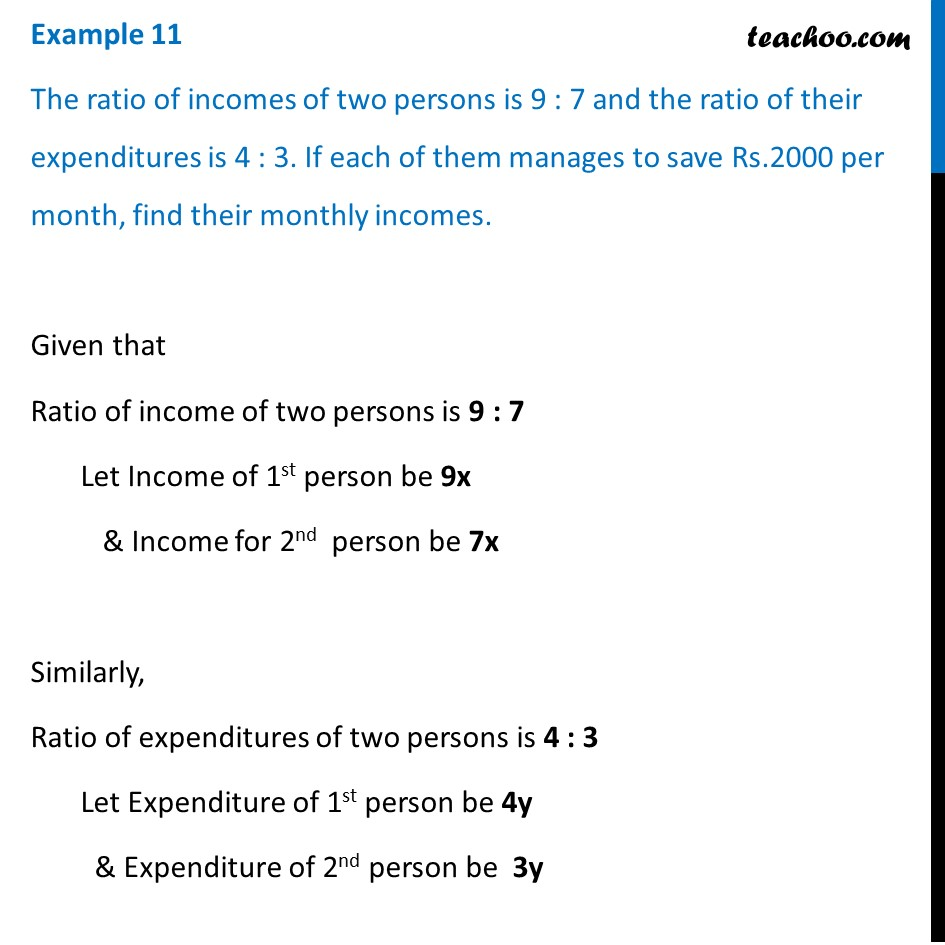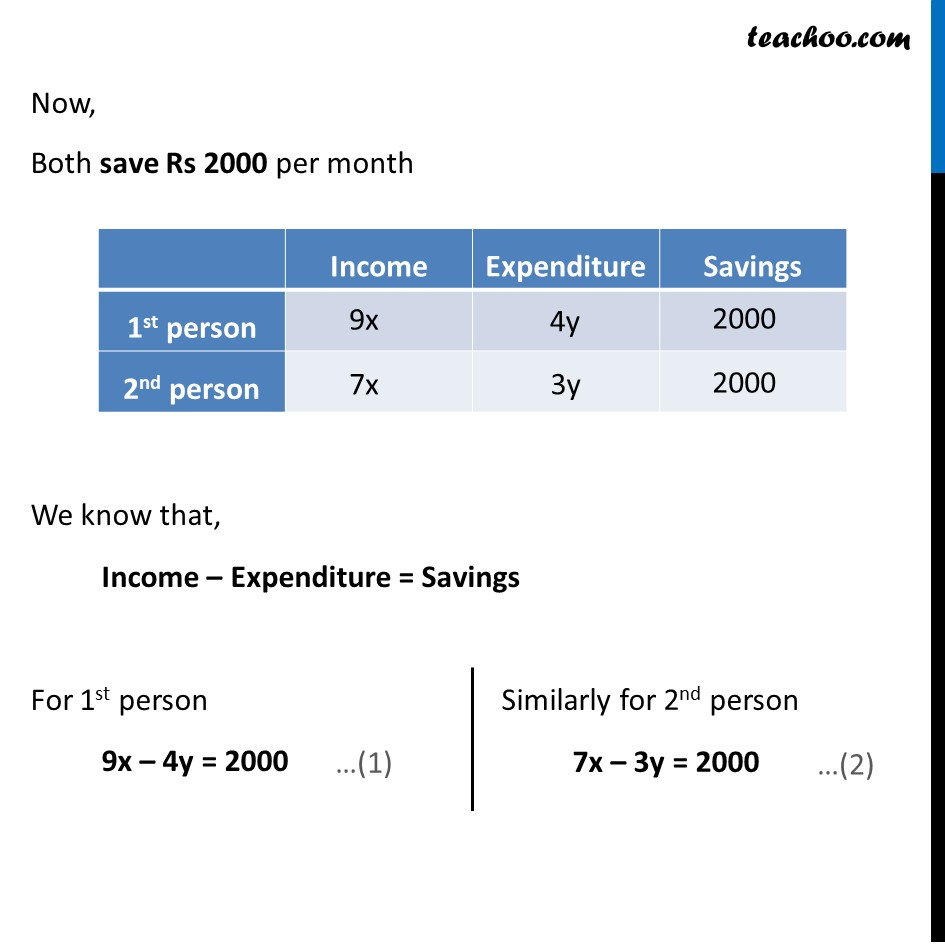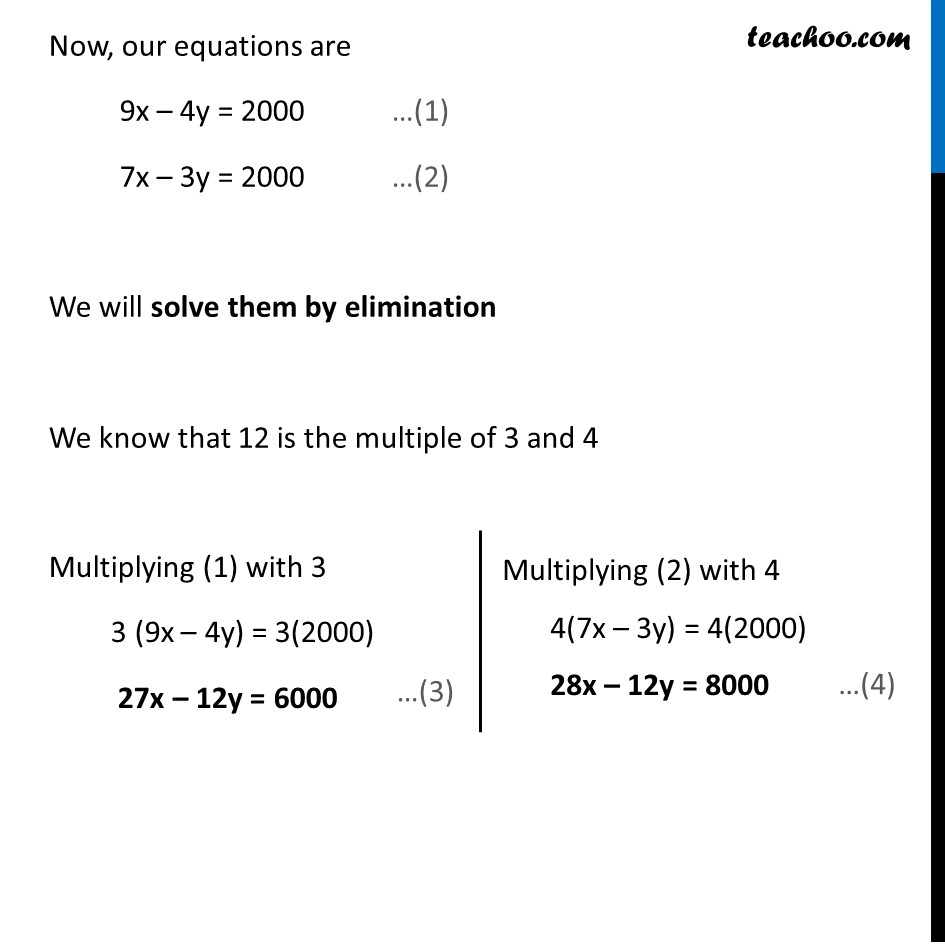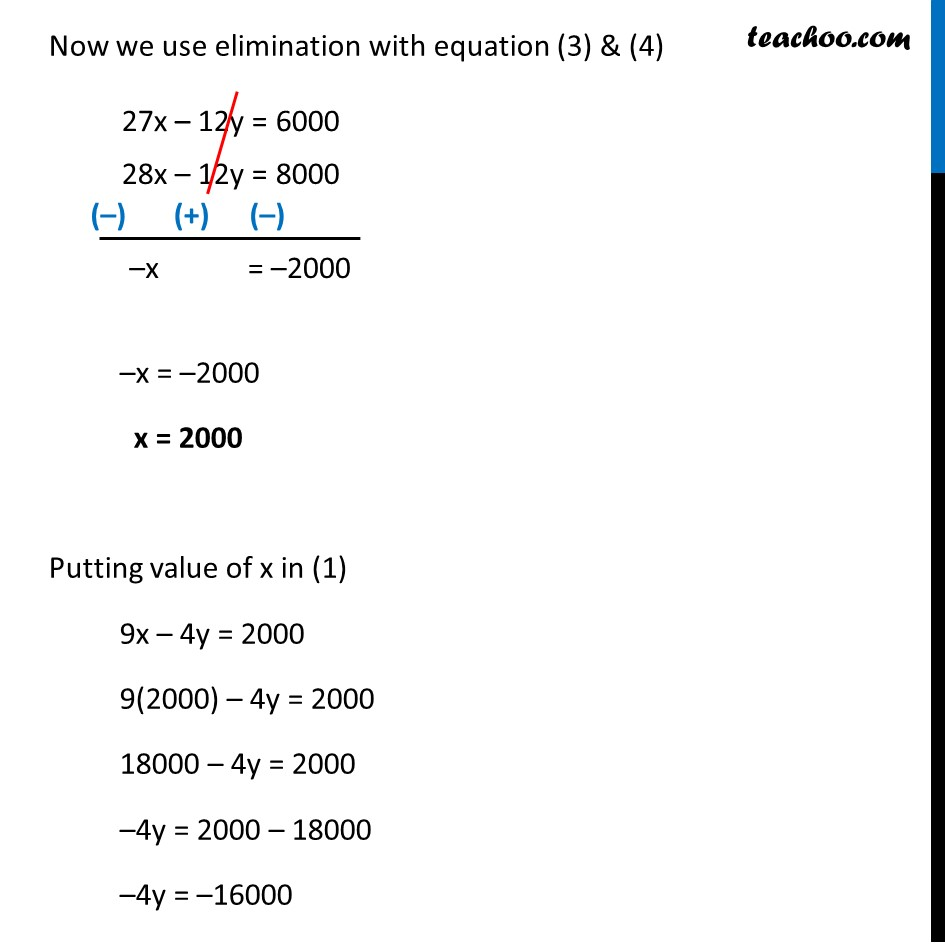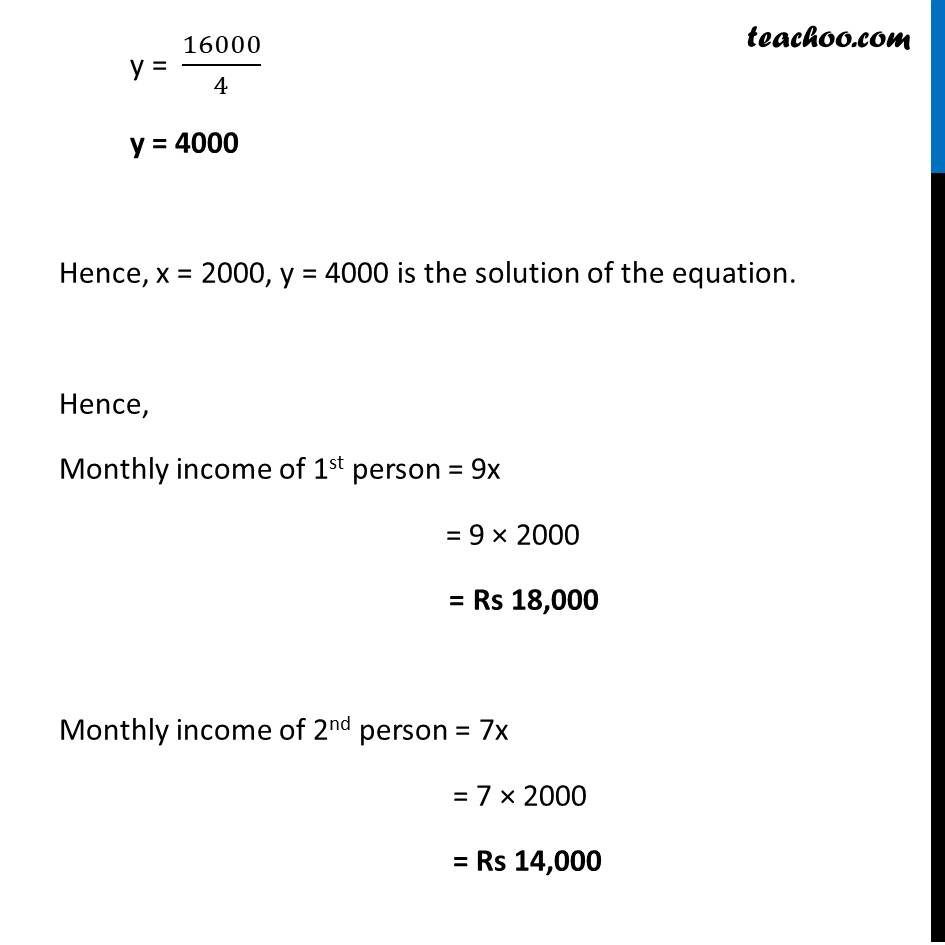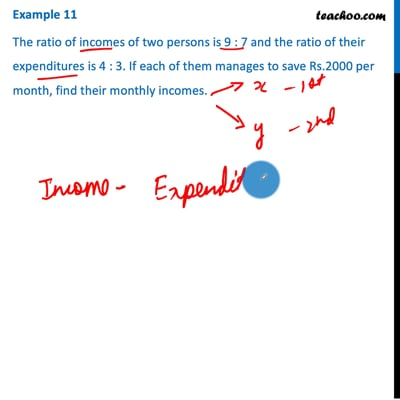This video is only available for Teachoo black users

### Transcript

Example 11 The ratio of incomes of two persons is 9 : 7 and the ratio of their expenditures is 4 : 3. If each of them manages to save Rs.2000 per month, find their monthly incomes. Given that Ratio of income of two persons is 9 : 7 Let Income of 1st person be 9x & Income for 2nd person be 7x Similarly, Ratio of expenditures of two persons is 4 : 3 Let Expenditure of 1st person be 4y & Expenditure of 2nd person be 3y Now, Both save Rs 2000 per month We know that, Income – Expenditure = Savings For 1st person 9x – 4y = 2000 Similarly for 2nd person 7x – 3y = 2000 Now, our equations are 9x – 4y = 2000 …(1) 7x – 3y = 2000 …(2) We will solve them by elimination We know that 12 is the multiple of 3 and 4 Multiplying (1) with 3 3 (9x – 4y) = 3(2000) 27x – 12y = 6000 Multiplying (2) with 4 4(7x – 3y) = 4(2000) 28x – 12y = 8000 Now we use elimination with equation (3) & (4) –x = –2000 x = 2000 Putting value of x in (1) 9x – 4y = 2000 9(2000) – 4y = 2000 18000 – 4y = 2000 –4y = 2000 – 18000 –4y = –16000 y = 16000/4 y = 4000 Hence, x = 2000, y = 4000 is the solution of the equation. Hence, Monthly income of 1st person = 9x = 9 × 2000 = Rs 18,000 Monthly income of 2nd person = 7x = 7 × 2000 = Rs 14,000